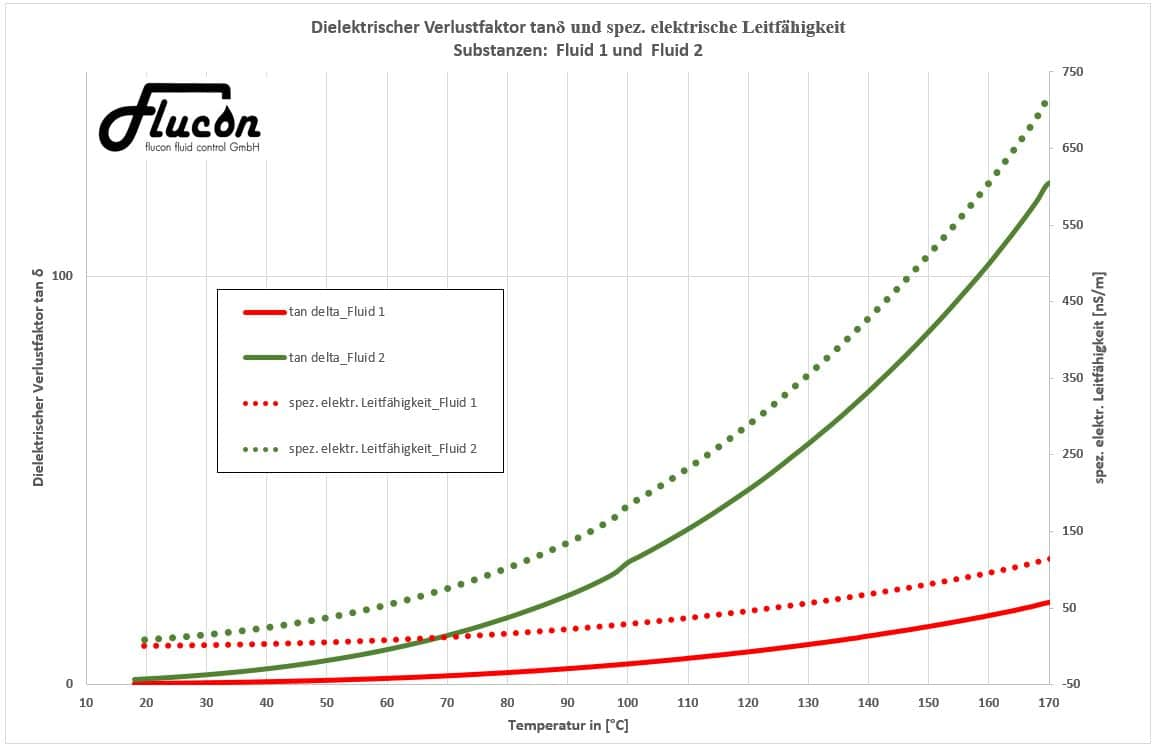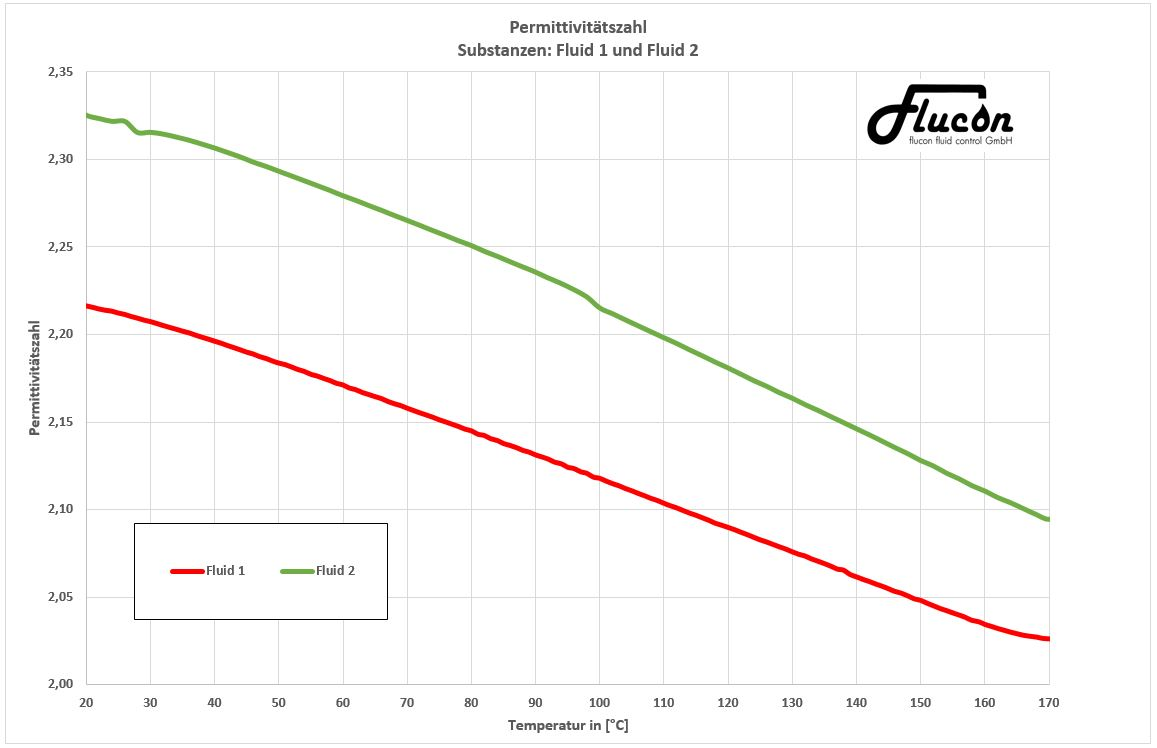# Dielectric fluid properties

## Specific electrical conductivity ϰ

The specific electrical conductivity, or conductivity, is a quantity which is often characterized by the symbol κ and indicates the ability of a substance to conduct electrical current. Its SI unit is S/m (Siemens per meter).

Our fluid impedance measuring system EPSILON+ delivers measured values in nS/m.

The suitability of a fluid to conduct electricity is determined by the number and mobility of the charge carriers within the liquid.

## Dielectric loss factor tan δ (DDF)

The dielectric loss factor, or dielectric dissipation factor (DDF) tan δ is a measure of the amount of energy that a substance in the alternating field absorbs and converts into heat loss. High dissipation factors are not suitable as insulating material in high frequency applications.

The loss factor is an indication of unwanted heat generation and thus provides information regarding the increased risk of thermal breakdown in transformer oils.

As can be seen in the following exemplary graph, both the specific electrical conductivity and the loss factor tan δ of a liquid are dependent on their temperature:

#### Exemplary results (measured with lab system EPSILON+)

(The Y-axis on the left shows the dielectric loss factor tan δ & the Y-axis on the right displays the specific electrical conductivity while the temperature is shown on the X-axis.)## Relative Dielectric / Permittivity / Dielectric Constant ε r

The relative dielectric, or permittivity (lat .: permittere = let through), of a substance is a measure of its permeability to electric fields.

The electric field constant ε 0, which corresponds to the permittivity of the vacuum, serves as a factor for determining the permittivity of a substance:

ε = ε 0 ε r

Here ε r is the substance-dependent relative permittivity. This is a dimensionless unit which is also temperature-dependent, as measurements with the EPSILON + display:

#### Exemplary results (measured with lab system EPSILON+)